Implements a Bloom filter. Which, as you may not know, is a space-efficient structure for storing a set. : Set « Collections Data Structure « Java

Implements a Bloom filter. Which, as you may not know, is a space-efficient structure for storing a set.

```

/*
* Created on 07-Mar-2005 by Ryan McNally
*/

//package com.ryanm.util.sets;

import java.util.Random;

/**
* Implements a Bloom filter. Which, as you may not know, is a
* space-efficient structure for storing a set.
*
* @author ryanm
*/
public class BloomFilter
{
/**
* We discard this number of randoms from the RNG when we set the
* seed, as the first few tend to be similar for similar seeds
*/
private final static int THROWAWAY_RANDOMS = 5;

/**
* The bitstring
*/
private boolean[] bitstring;

/**
* We use the output of the RNG as a hash function
*/
private final Random hash = new Random( 6381273189l );

/**
* The number of hash functions to use
*/
private int hashCount;

/**
* A magic number used to compute what size a bloom filter should
* be. Bloom filters should be ( members * hashes ) / bloomMagic
* long
*/
public static final double bloomMagic = -Math.log( 0.5 ) / Math.log( 2 );

/**
* Constructs a new Bloom filter
*
* @param filterSize
*           The size of the filter's bitstring
* @param hashCount
*           The number of hash functions to use when inserting
*           into and querying the filter
*/
public BloomFilter( int filterSize, int hashCount )
{
bitstring = new boolean[ filterSize ];
this.hashCount = hashCount;
}

/**
* Builds a Bloom filter with the optimum length
*
* @param members
*           The members to enter into the filter
* @param hashCount
*           The number of hashes to use
* @return An optimally-sized filter that contains the specified
*         elements
*/
public static BloomFilter buildFilter( int[] members, int hashCount )
{
int filterLength = ( int ) ( members.length * hashCount / BloomFilter.bloomMagic );

filterLength = Math.max( filterLength, 1 );

BloomFilter filter = new BloomFilter( filterLength, hashCount );

for( int i : members )
{
filter.insert( i );
}

return filter;
}

/**
* Inserts an element into this filter
*
* @param i
*           The element to insert
*/
public void insert( int i )
{
int[] indices = generateIndices( i );

for( int j = 0; j < indices.length; j++ )
{
bitstring[ indices[ j ] ] = true;
}
}

/**
* Tests if this filter contains an element
*
* @param i
*           The element to test for
* @return true if the filter may contain the element, false if it
*         definitely does not
*/
public boolean contains( int i )
{
int[] indices = generateIndices( i );

for( int j = 0; j < indices.length; j++ )
{
if( !bitstring[ indices[ j ] ] )
{
return false;
}
}

return true;
}

/**
* Clears the filter of all elements
*/
public void clear()
{
for( int i = 0; i < bitstring.length; i++ )
{
bitstring[ i ] = false;
}
}

/**
* Generates the indices for a given element
*
* @param i
*           The element
* @return An array of the indices to set or check
*/
private int[] generateIndices( int i )
{
// prepare the rng
hash.setSeed( i );
for( int j = 0; j < THROWAWAY_RANDOMS; j++ )
{
hash.nextInt( bitstring.length );
}

// generate the indices
int[] indices = new int[ hashCount ];
for( int j = 0; j < indices.length; j++ )
{
indices[ j ] = hash.nextInt( bitstring.length );
}

return indices;
}

/**
* Sets the length of the bit string in this filter. Note that this
* also has the effect of clearing all entries
*
* @param size
*           The new size of the bit string
*/
public void setSize( int size )
{
bitstring = new boolean[ size ];
}

/**
* Gets the size of this filter's bit string
*
* @return the size of this filter's bitstring
*/
public int getSize()
{
return bitstring.length;
}

/**
* Sets the number of hashes that this filter will use. Note that
* this also has the effect of clearing all entries
*
* @param hashes
*           The number of hashes to use.
*/
public void setHashes( int hashes )
{
hashes = Math.max( 0, hashes );

hashCount = hashes;

clear();
}

/**
* Gets the number of hashes used in this filter
*
* @return the number of hashes
*/
public int getHashes()
{
return hashCount;
}

/**
* Gets the number of bits that have been set in this filter
*
* @return The number of bits that are set to 1 in this filter
*/
public int bitsSet()
{
int count = 0;
for( int i = 0; i < bitstring.length; i++ )
{
if( bitstring[ i ] )
{
count++;
}
}

return count;
}

/**
* Returns the saturation level of this filter. When all bits are
* set, saturation is 1.0, when no bits are set, saturation is 0.0.
* You get the idea
*
* @return The saturation level
*/
public float saturation()
{
return ( float ) bitsSet() / ( float ) bitstring.length;
}

/**
* Clones this filter's bitstring
*
* @return a new boolean array, with the same bits set as in this
*         filter
*/
public boolean[] cloneFilter()
{
boolean[] array = new boolean[ bitstring.length ];

System.arraycopy( bitstring, 0, array, 0, array.length );

return array;
}

/**
* Calculates the hamming distance between this filter and the
* supplied array. ie: the number of bits that do not correspond.
* The array must be the same size as this filter.
*
* @param b
* @return the hamming distance, or -1 if the two arrays are not
*         the same size
*/
public int hammingDistance( boolean[] b )
{
if( b.length == bitstring.length )
{
int count = 0;

for( int i = 0; i < b.length; i++ )
{
if( b[ i ] != bitstring[ i ] )
{
count++;
}
}

return count;
}

return -1;
}

}

```

Related examples in the same category

 1 Set, HashSet and TreeSet 2 Things you can do with Sets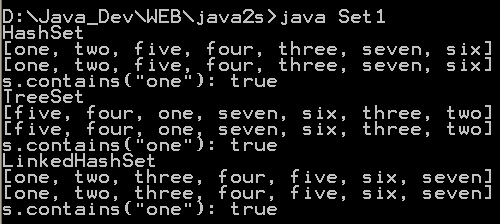3 Set operations: union, intersection, difference, symmetric difference, is subset, is superset 4 Set implementation that use == instead of equals() 5 Set that compares object by identity rather than equality 6 Set union and intersection 7 Set with values iterated in insertion order. 8 Putting your own type in a Set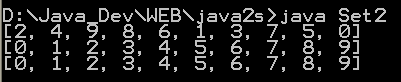9 Use set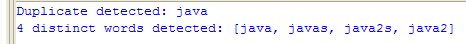10 Another Set demo 11 Set subtraction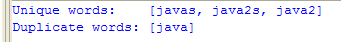12 Working with HashSet and TreeSet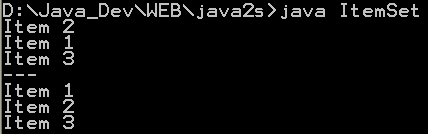13 TreeSet Demo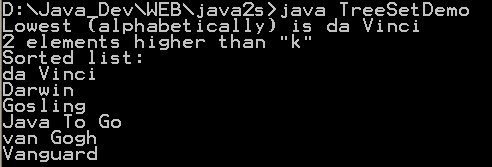14 Show the union and intersection of two sets 15 Demonstrate the Set interface 16 Array Set extends AbstractSet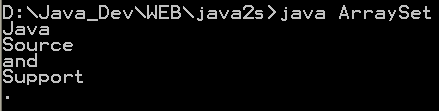17 Sync Test 18 Set Copy 19 Set and TreeSet 20 Tail 21 What you can do with a TreeSet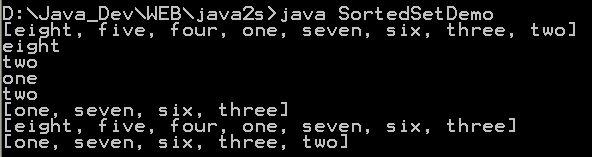22 Remove all elements from a set 23 Copy all the elements from set2 to set1 (set1 += set2), set1 becomes the union of set1 and set2 24 Remove all the elements in set1 from set2 (set1 -= set2), set1 becomes the asymmetric difference of set1 and set2 25 Get the intersection of set1 and set2, set1 becomes the intersection of set1 and set2 26 Extend AbstractSet to Create Simple Set 27 Int Set 28 One Item Set 29 Small sets whose elements are known to be unique by construction 30 List Set implements Set 31 Converts a char array to a Set 32 Converts a string to a Set 33 Implements the Set interface, backed by a ConcurrentHashMap instance 34 An IdentitySet that uses reference-equality instead of object-equality 35 An implementation of the java.util.Stack based on an ArrayList instead of a Vector, so it is not synchronized to protect against multi-threaded access. 36 A thin wrapper around a List transforming it into a modifiable Set. 37 A thread-safe Set that manages canonical objects 38 This program uses a set to print all unique words in System.in 39 Indexed Set 40 An ObjectToSet provides a java.util.Map from arbitrary objects to objects of class java.util.Set. 41 Sorted Multi Set 42 Fixed Size Sorted Set 43 Set operations 44 A NumberedSet is a generic container of Objects where each element is identified by an integer id. 45 Set which counts the number of times a values are added to it. 46 Set which counts the number of times a values are added to it and assigns them a unique positive index. 47 Indexed Set 48 A set acts like array. 49 Implementation of disjoint-set data structure 50 Call it an unordered list or a multiset, this collection is defined by oxymorons